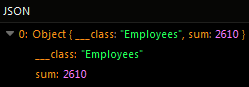# Retrieving Unique Objects¶

Unique objects can be retrieved from a column of the requested table by using the `distinct` property in the invocation. The `distinct` parameter is a boolean value which defaults to `false`.

The `distinct` property can be also used with Aggregate Functions such as AVERAGE, COUNT, and SUM. When applied to an aggregate function, the `distinct` property ensures that only distinct values are considered in the calculation, eliminating any duplicates from the result.

For example, when using the distinct property with the `COUNT` function, it counts only the unique occurrences of a particular column, disregarding any repetitions. Similarly, when combined with `AVERAGE` or `SUM`, the `distinct` property ensures that only distinct values are considered in the calculation, providing accurate results based on unique values within the dataset.

Consider the following data table called `Employees`: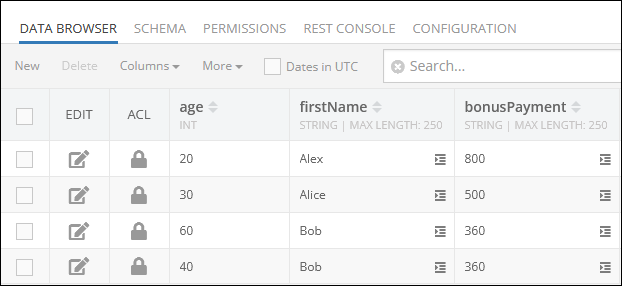This table contains the column `firstName`, and by passing the `true` value to the `distinct` property when calling this method, only unique values will be returned in the response:

Important

Make sure to replace xxxx in the domain name in the sample requests below to the one assigned to your application.

``````GET https://xxxx.backendless.app/api/data/Employees?props=firstName&distinct=true
``````

The query returns the following result which contains only unique names:

``````[
{
"___class": "Employees",
"firstName": "Alex"
},
{
"___class": "Employees",
"firstName": "Alice"
},
{
"___class": "Employees",
"firstName": "Bob"
}
]
``````

### Aggregate Functions¶

The `distinct` parameter can be also used with such aggregate functions as:

All examples presented further use sample data from the `Employees` data table presented in the beginning of this topic.

#### Average¶

Suppose you need to calculate an average value for a set of objects, but you want to skip identical records. The following example will retrieve only unique values from the `age` column and calculate the average age of all employees.

Important

Make sure to replace xxxx in the domain name in the sample requests below to the one assigned to your application.

``````GET https://xxxx.backendless.app/api/data/Employees?props=Avg(distinct age)
``````

#### Count¶

The example below will count only unique values in the `firstName` column and return an integer value in the response:

``````GET https://xxxx.backendless.app/api/data/Employees?props=Count(distinct firstName)
``````

#### Sum¶

The example below will calculate the sum of unique values in the `bonusPayment` column and return an integer value in the response:

``````GET https://xxxx.backendless.app/api/data/Employees?props=Count(distinct bonusPayment)
``````

### Codeless Reference¶

Consider the following data table called `Employees`: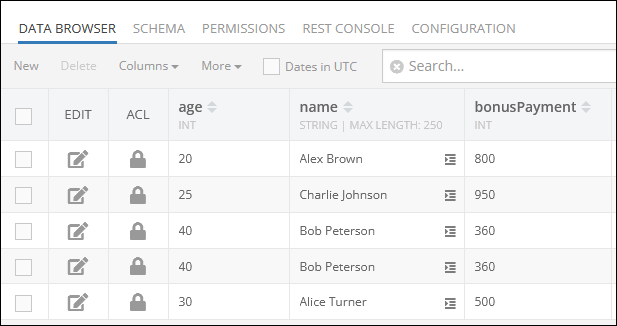#### AVERAGE¶

Suppose you need to calculate the average age of your employees, while excluding duplicates. As shown in the data table schema above, there are two identical records with the name `"Bob Peterson"`. that have the value of `40` in the `"age"` column.

The example below returns the average age only for unique records stored in the `Employees` data table: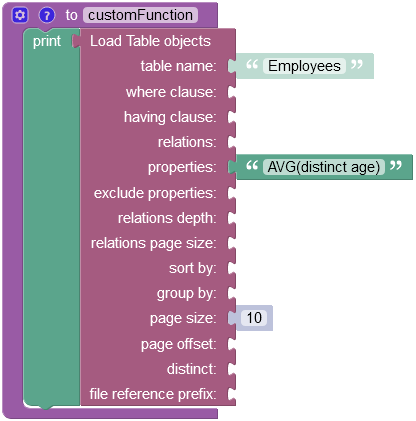The operation returns the following result in the response: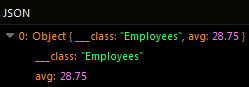#### COUNT¶

Suppose you need to count the number of employees, while excluding duplicates. Considering the data table schema provided above, there are two identical entities with the name `"Bob Peterson"`.

The example below counts the total number of unique employee `names` in the `Employees` data table: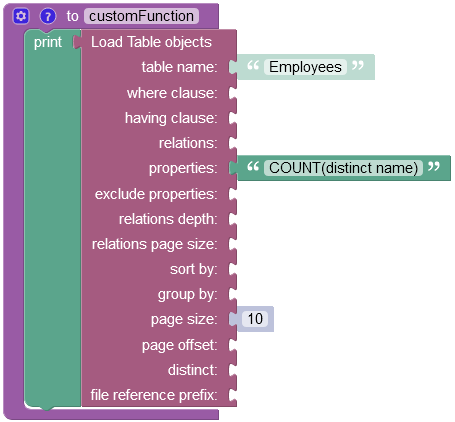The operation returns the following result in the response: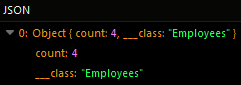#### SUM¶

Suppose you need to calculate the sum of all bonus payments, while excluding duplicates. Considering the data table schema provided above, there are two identical records that have the `360` value stored in the `"bonusPayment"` column:

The example below excludes the duplicate entity and calculates the sum of unique values: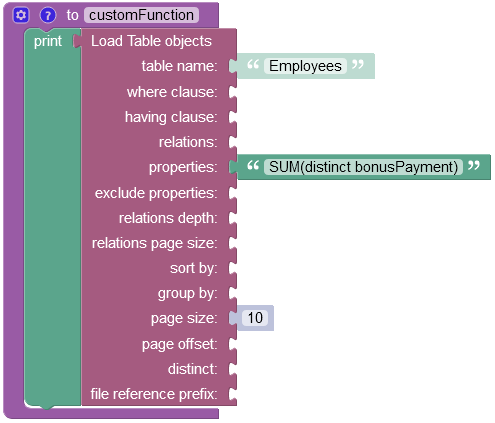The operation returns the following result in the response: